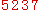下的实例中使用3个表 （TABLE） A(R1,R2,R3,R4,R5,C1,C2,B2) B(R1,B1,B2) C(R1,C1,C2,B2),括号中即为表中的字段。

一、使用特定表中的索引。

有时候一个表中的数据量很大时候，索引是非常非常的重要，何为索引？我来举个例子，你一听就能明白，比如：你想搜索我的电脑中一个名字为FILE.TXT文件，如果不知道该文件所在的目录（没有创建索引）情况下，基本上要扫描我的电脑中的所有盘符里的文件，如果你的硬盘中的有很多文件（相当一个表中有很多的数据），基本上是一个全盘扫描的过程，这时如果你告诉搜索，你的文件就在D盘的TEST目录下，这样来搜索速度将会大大的提高，给文件定位就相当一个索引。

好了，知道索引是干什么之后，下面来看看怎么走正确的索引，才能达到最高效。假如A表中有两个索引IDX_R1 INDEX(R1,R2,R4,R5),IDX_R3 INDEX(R1,R3,R4,R5) 现在需要做这样的操作，显示表中的条件值是R1 = 1,R3 = 3,R4 =4的所有内容。通常我们会写出下面的查询语句。
select * from A where R1 =1 and R3 =3 and R4;

select /*+ index(R1,IDX_R3) */* from A where R1 =1 and R3 =3 and R4;

SELECT  B.R1,B.B1,B.B2 FROM A,B WHERE A.R1 = B.R1;
优化为：
SELECT  B.R1,B.B1,B.B2 FROM B WHERE EXISTS(SELECT 1 FROM A WHERE A.R1 = B.R1);
如果你在WINDOWS的ORACLE的平台可以使用PL/SQL中的执行计划（EXPLAIN PLAN），看一下相应的执行时间进行验证。

SELECT A.R2 FROM A WHERE B.B2 = 3 AND 2< SELECT COUNT(1) FROM B WHERE B.R1 = A.R1;  //效率较低

SELECT A.R2 FROM A WHERE 2< SELECT COUNT(1) FROM B WHERE B.R1 = A.R1 AND B.B2 = 3;  //效率较高

//1
SELECT SUM(Nvl(C2,0)) FROM C WHERE R1 = 12 AND C1 = 22 ;
//2
SELECT SUM(Nvl(C2,0)) FROM C WHERE R1 = 12 AND C1 = 33 ;

SELECT SUM(DECODE(C1,22,C2,33,C2,0) FROM C WHERE R1 =12 AND C1 IN(22,33);

SELECT * FROM A WHERE TO_CHAR(R5,'YYYYMM') = '200804'

SELECT * FROM A WHERE R5 = TO_DATE('200804','YYYYMM');代替

UPDATE A
SET C2 = (SELECT MAX(C2) FROM C),
B2 = (SELECT MAX(B2) FROM C)
WHERE R1 = 1000;

UPDATE A
SET (C2, B2)
= (SELECT MAX(C2) , MAX(B2)
FROM C)
WHERE R1 = 1000;

//低效:
SELECT DISTINCT C.R1,C.B2
FROM A,C
WHERE A.R1 = C.R1 ;

//高效:
SELECT C.R1,C.B2
FROM C
WHERE EXISTS ( SELECT ‘X’
FROM A
WHERE A.R1 = C.R1);

EXISTS 使查询更为迅速,因为RDBMS核心模块将在子查询的条件一旦满足后,立刻返回结果.